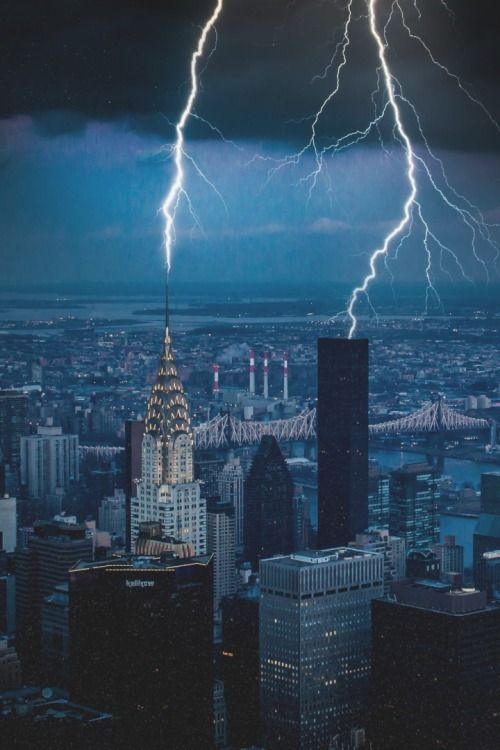# How to count the frequency of lightning strikes into a building?

23.12.16

If we treat this matter formally, then it is not such a complicated task to calculate how often the lightning strikes a house. For that, we would need the area that collects lighting and their density. We multiply all these quantities and get a precise calculation of strike frequency per year. But these are all theories, and what do we have in practice?

In reality, the density is less than one and is represented by a fractional number that is physically meaningless. To get a sensible response, we should conditionally equalize the density of strikes to one: Tlightn ≈ 1/NM. All existing reference books register the density per 1 sq.km per year, and thus the characteristics of Tlightn is calculated in years. For instance, if nМ = 0.004, then it is assumed that the frequency of lightning strikes into a building (1:0.04) will be once per 25 years on average.The word “average” has a special meaning. That does not at all mean that lightning will strike 25 years after the building has been erected. That may occur even much earlier, even in the same year. Although another outcome is possible: there might be no lightning strike into the object in 90 years. The calculated time period is an average. To make a more realistic calculation of the frequency of lightning strikes, it is necessary to watch a huge number of buildings of the same type for a very long period of time. For details on how to calculate the collection area and the specific density in order to calculate the frequency of lightning strikes into a building, read our new useful article!